# Capillarity

## Capillarity:

Capillarity is a phenomenon of rise or fall of liquid surface relative to the adjacent general level of liquid. This phenomenon is due to the combined effect of cohesion and adhesion of liquid particle. The rise of liquid level is known as capillary rise whereas the fall of liquid surface is known as capillary depression. It is expressed in terms of cm or mm of liquid.

The magnitude of capillarity is dependent upon

• Diameter of tube.
• Specific weight of liquid.
• Surface tension of liquid.

Let d be the diameter of the glass tube and θ is the contact angle between liquid and glass tube. The water shall rise in the tube till vertical component of surface tension equals the weight of the water column.

The surface tension force acting around the circumference of the tube = σ x πd.

The vertical component of this force = σ x πd x Cosθ ————(i)

Rise of the water in the tube (i.e. height) = h

The weight of the liquid column of height h in the tube = Area of the tube x h x Specific weight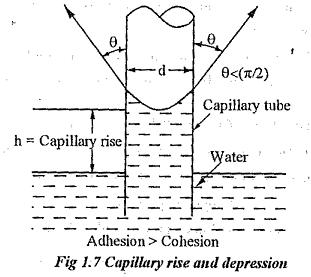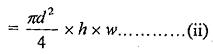Equating the equations (i) and (ii)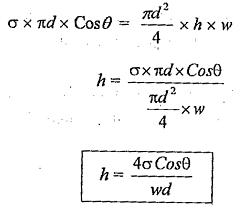Note: For water and glass tube interface, θ= 0.

So, the capillary rise of water in the glass tube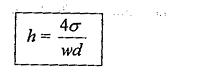For Mercury and glass tube interface, θ  ≈ 140°Cosθ value is negative, and hence his also negative. Therefore, the capillary depression is caused.

The glass tube used for pressure measurement should have sufficient diameter (more than 12mm) to eliminate the influence of capillary action.

### Compressibility and Bulk Modules(K):

Bulk modulus (K) is defined as the ratio of change in pressure to the rate of change of volume due to the change in pressure.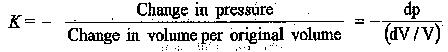Where ‘— ‘ ve sign indicates decrease in volume with increase in pressure. It can also be expressed in terms of change of density,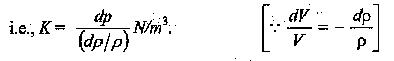The bulk modulus for liquids depends on both pressure and temperature. The value increases with pressure; as dV will be lower at higher pressures for the same value of dp. The bulk modulus generally increases with temperature.

Compressibility is the property by virtue of fluid undergoes a change in volume wider the action of external pressure. It is the reciprocal of bulk modulus.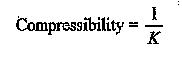It decreases with increase in pressure as dV increases with increase in pressure.

Updated: October 27, 2017 — 8:58 pm